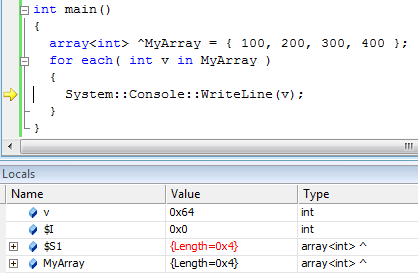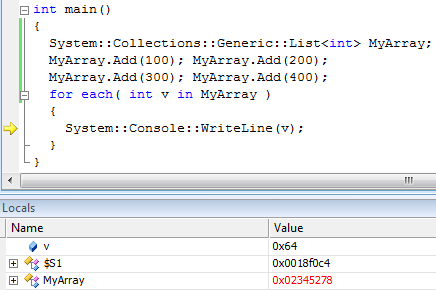# The mystic variable “\$I” during for each

A poster in the german C/C++ forum asked if there is an index available while using a for each loop. He accidently saw in the debug-window a variable called “\$I”.
And indeed: There is a “hidden” variable “\$I” which can be used inside the for each loop. This variable is the number of the loop-iteration.

Here is a simple example:

```int main()
{
array<int> ^MyArray = { 100, 200, 300, 400 };
for each( int v in MyArray )
{
System::Console::WriteLine(v.ToString() + " " + \$I);
}
return 0;
}
```

As you can see, it uses a variable “\$I” which was never decalred!
And it will output the following:

```100 0
200 1
300 2
400 3```

If you debug a normal for each loop and take a look in the “local-watch-window”, you will see the following variables, while you are inside the for each loop:You can see two “hidden” variables “\$I” and “\$S1”. And if you step through the loop, you will see that the “\$I” variable is incremented for each iteration. “\$S1” is a reference to the array.

If you dig further into this issue, you will find out, that for each and the normal “for” loop will result in the identical IL code! This is also true for C#!
FOREACH Vs. FOR (C#)
To foreach or not to foreach that is the question.

As we can see, the “\$I” variable is just a side-effect of the for each loop. It is a compiler generated variable which is used to transform the “for each” into a normal for-loop!

Of course, this is only true in special cases like arrays.
If you have a list which only implements the “IEnumerable” interface and is not an array (like “System::Collections::Generic::List”), then the mistic variable “\$I” is gone, because now the compiler uses the “IEnumerable” interface to gbuild a “real” “for each” loop:In this case “\$I” is gone but “\$S1” is still there. And “S1” is the enumerator of the list (in this case

`System::Collections::Generic::List::Enumerator<int>`

).

The conclusion is:
Do not rely on the compiler generated variable “\$I”, and do not use “for each” if you need a index-variable, just use a normal for loop.